## Create a marimekko chart in Excel

Marimekko chart is also known as Mosaic chart, which can be used to visualize data from two or more qualitative variables. In a Marimekko chart, the column widths show one set of percentages, and the column stacks show another set of percentages.

The below Marimekko chart demonstrates the sales of Drink, Food and Fruit in a company from 2016 to 2020. As you can see, the column widths show the size of market segment for Drink, Food and Fruit in a year, and each segment in the column shows the sales for a certain category.

This tutorial will demonstrate the steps to create a Marimekko chart in Excel step by step.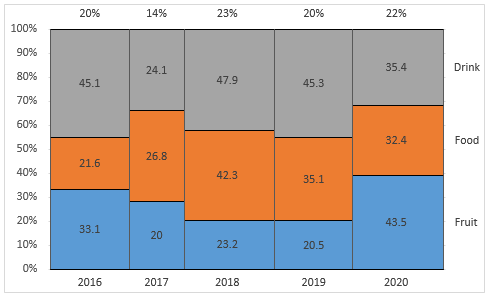Create a Marimekko chart in Excel

#### Create a Marimekko chart in Excel

Supposing you want to create a Marimekko chart based on data as the below screenshot shown, you can do as follows to get it down.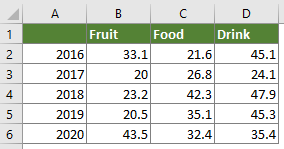##### Part1: Create an intermediate data table

1. Create an intermediate data table based on the original data as follows.

The first column of the intermediate data table

As the below screenshot shown, values in the first helper column represent the position where each column ends on the X axis. Here we specify the minimum of the X-axis as 0 and the maximum as 100, so the column starts from 0 and ends with 100. You can do as follows to get the data between the minimum and maximum.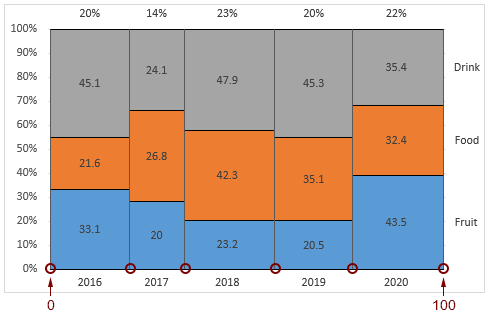> Enter number 0 into the second cell (A9 in this case) of the column (keep the column header blank);
> Enter the below formula into cell A10 and press the Enter key. Repeat the same formula in A11;
=SUM(\$B\$2:\$D\$2)/SUM(\$B\$2:\$D\$6)*100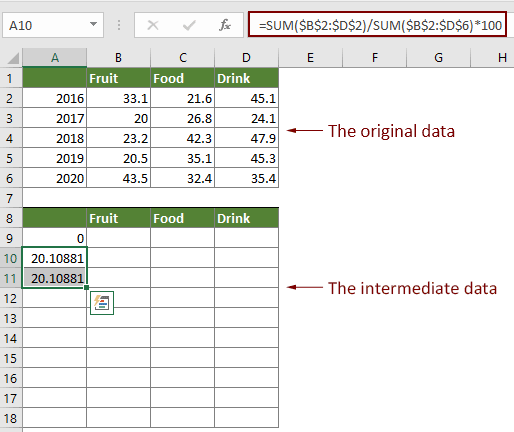> Enter the below formula into A12 and press the Enter key. Repeat this formula in A13 to get the same result.
=SUM(\$B\$3:\$D\$3)/SUM(\$B\$2:\$D\$6)*100+A10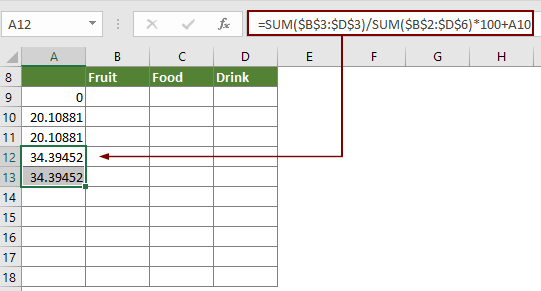> Enter the below formula into A14 and then press the Enter key. Repeat this formula to A15 to get the same result.
=SUM(\$B\$4:\$D\$4)/SUM(\$B\$2:\$D\$6)*100+A12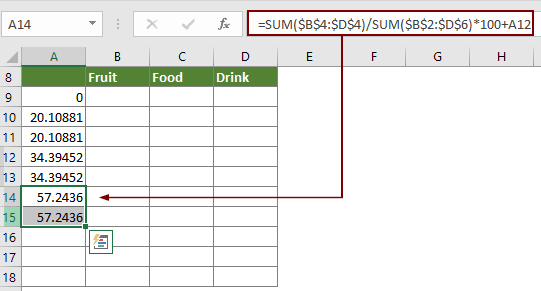> Enter the below formula into A16 and then press the Enter key. Apply this formula to A17 to get the same result.
=SUM(\$B\$5:\$D\$5)/SUM(\$B\$2:\$D\$6)*100+A14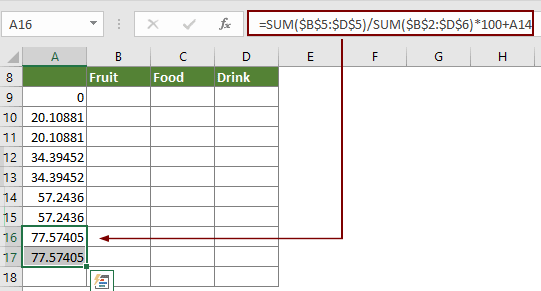> Enter number 100 into the last cell (A18). And finally the first column is complete.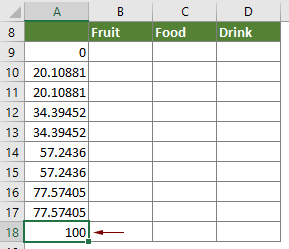The other columns of the intermediate data table

Values in these columns represent the height for series in each stacked column. See screenshot: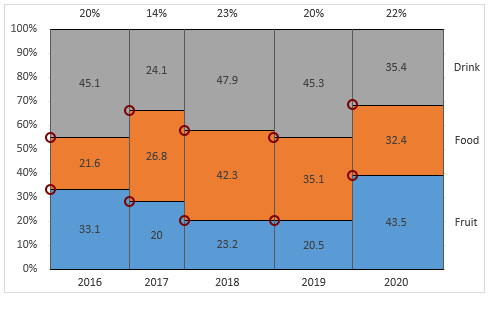> Enter the same headers as the original data into the other three columns;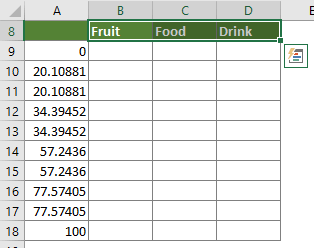> In the second column, enter the below formula into the first blank cell (B9) and then press the Enter key. Select this result cell, drag the AutoFill Handle right to the next two cells and then down to the next row.
=B\$2/SUM(\$B\$2:\$D\$2)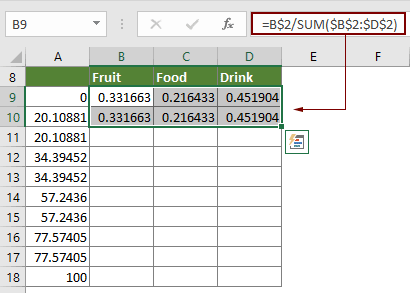> Enter the below formula into B11 and then press the Enter key. Select this result cell, drag the AutoFill Handle right to the next two cells and then down to the next row.
=B\$3/SUM(\$B\$3:\$D\$3)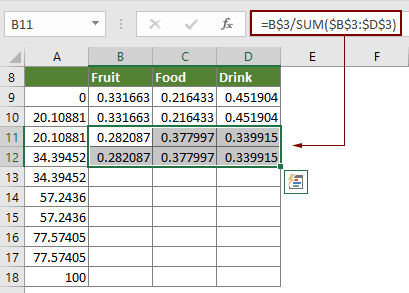> Go on entering the below formula into B13 and then press the Enter key. Select this result cell, drag the AutoFill Handle right to the next two cells and then down to the next row.
=B\$4/SUM(\$B\$4:\$D\$4)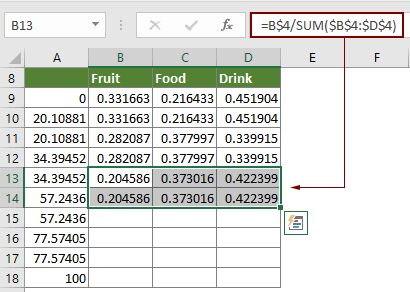> Enter the below formula into B15 and then press the Enter key. Select this result cell, drag the AutoFill Handle right to the next two cells and then down to the next row.
=B\$5/SUM(\$B\$5:\$D\$5)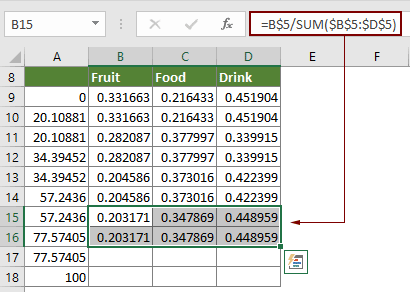> Enter the below formula into B17 and then press the Enter key. Select this result cell, drag the AutoFill Handle right to the next two cells and then down to the next row.
=B\$6/SUM(\$B\$6:\$D\$6)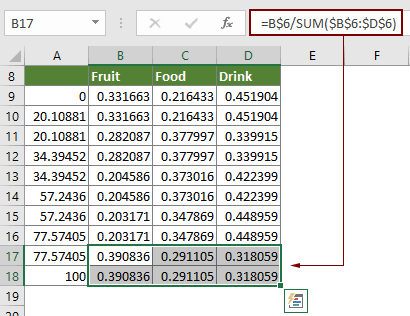##### Part2: Insert a stacked area chart based on the intermediate data and format it

2. Select the whole intermediate data table, click Insert > Line Chart or Area Chart > Stacked Area.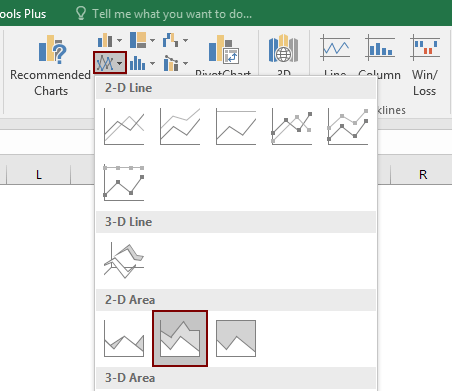3. Right click the X-axis in the chart and select Format Axis from the right-clicking menu.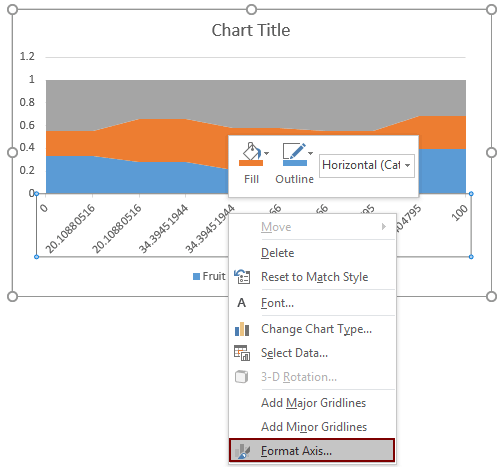4. In the Format Axis pane, select the Date axis option under the Axis Options tab.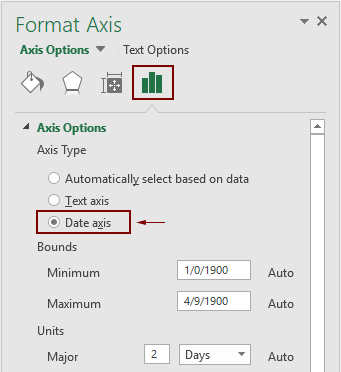5. Keep the X-axis selected and then press the Delete key to remove it from the chart.

Then the chart is displayed as follows.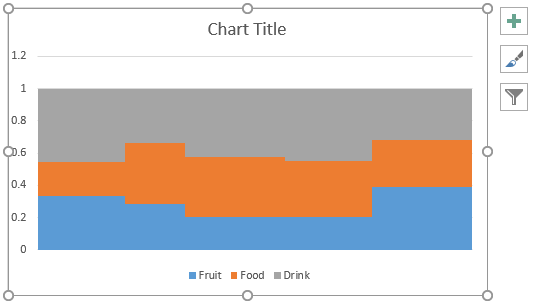6. Right click the Y-axis and select Format Axis from the context menu.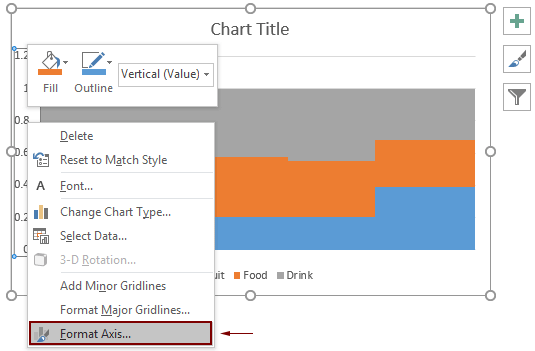7. In the Format Axis pane, please configure as follows.

7.1) In the Axis Options section, change the Maximum box to 1;
7.2) In the Number section, select Percentage from the Category drop-down list, and then change the Decimal places to 0.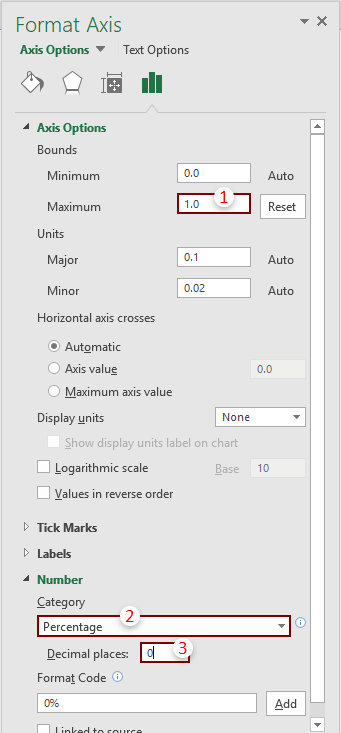Now the chart is displayed as follows.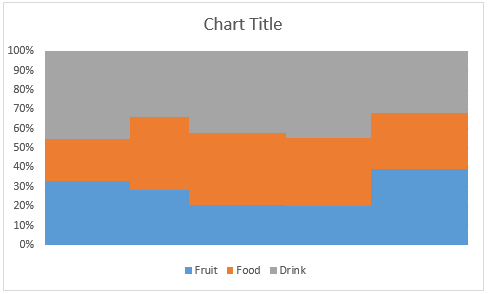8. Now we need to add borders to show the occupation of each data in a series. Please do as follows.

8.1) Select the top gray area (the Drink series in this case), click the Chart Elements button, and then check the Error Bars box.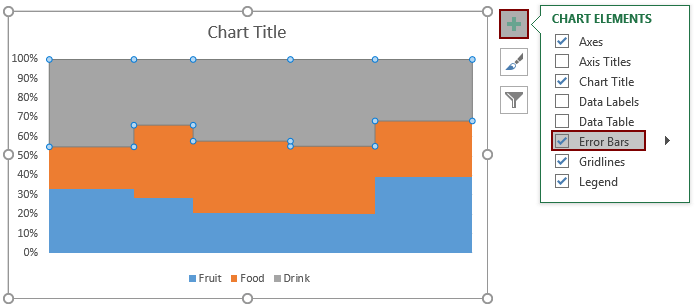8.2) Go to the Format Data Series pane, click the Series Option drop-down arrow, and then select Series “Drink” Y Error Bars from the list to select the error bars you have added just now.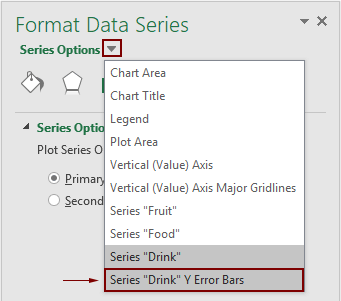8.3) Under the Error Bar Options tab, you need to:
• Select Minus in the Direction section;
• Select No Cap in the End Style section;
• Select the Percentage option and enter 100 into the text box in the Error Amount section.
•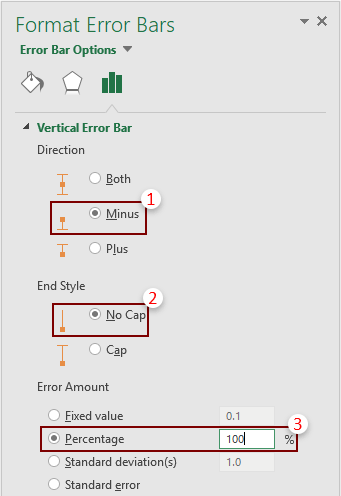8.4) Select the gray bar (the Drink series), and then go to the Format Data Series pane to configure as follows.
• Click the Fill & Line icon;
• In the Border section, select Solid line;
• Select the black color in the Color drop down list;
• Change the Width to 1pt.
•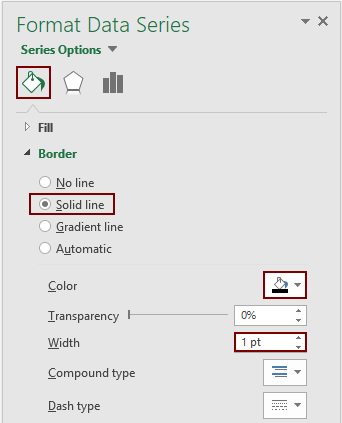Now the chart is displayed as the below screenshot shown.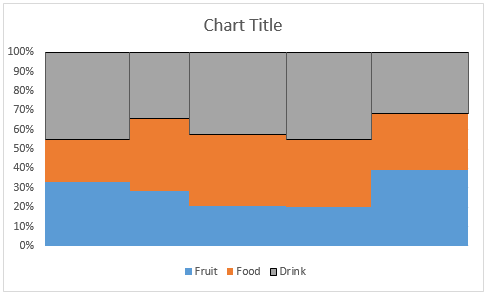9. Repeat the operations in step 8 to add dividers to other segments. And finally the chart is shown as below.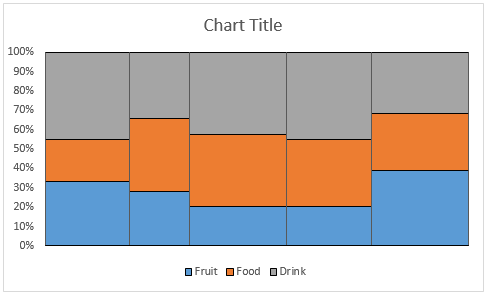##### Part3: Specify the X-axis values of the Marimekko chart

10. Now you need to calculate the middle value for each column and display the subcategory values (the first column data of the original data range) as the X-axis values.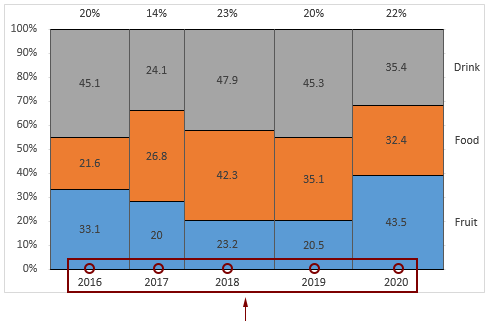Two helper rows are needed in this section, please apply the below formulas to handle it.

10.1) In the first cell (here I select A22) of the first row, enter the below formula into it and press the Enter key to get the result.
=A11/2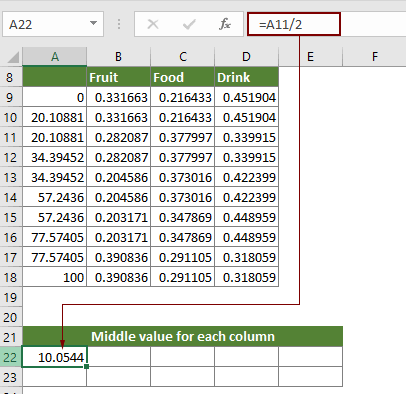10.2) In the second cell of the first row, apply the below formula.
=(A13-A11)/2+A11
10.3) In the third cell of the first row, apply the below formula.
=(A15-A13)/2+A13
10.4) In the fourth cell of the first row, apply the below formula.
=(A17-A15)/2+A15
10.5) In the fifth cell of the first row, apply the below formula.
=(A18-A17)/2+A17
10.6) Enter number 0 into each cell of the second row. And finally this helper range is displayed as the below screenshot shown.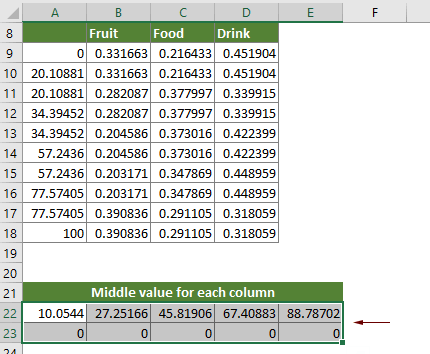11. Right click the chart and click Select Data in the right-clicking menu.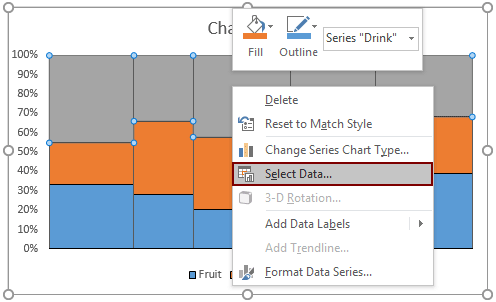12. In the opening Select Data Source dialog box, click the Add button.

13. Then an Edit Series dialog box pops up, please select the cells containing the 0 values in the Series value box, and then click the OK button.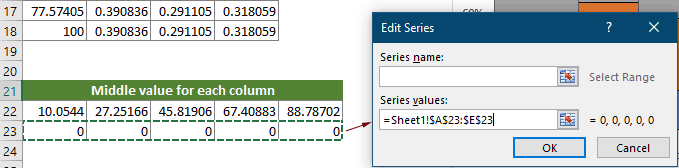14. When it returns to the Select Data Source dialog box, you can see a new series (Series4) is created, click the OK button to save the changes.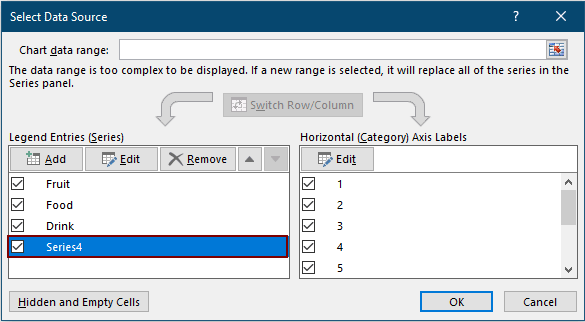15. Right click the chart and select Change Series Chart Type.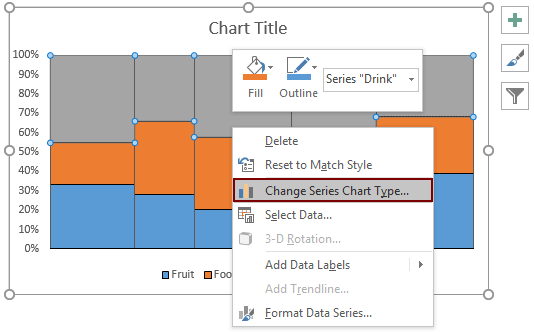16. In the Change Chart Type dialog box, select the chart type “Scatter with Straight Lines and Markers” for the Series4 in the Choose the chart type and axis for your data series box. And then click OK.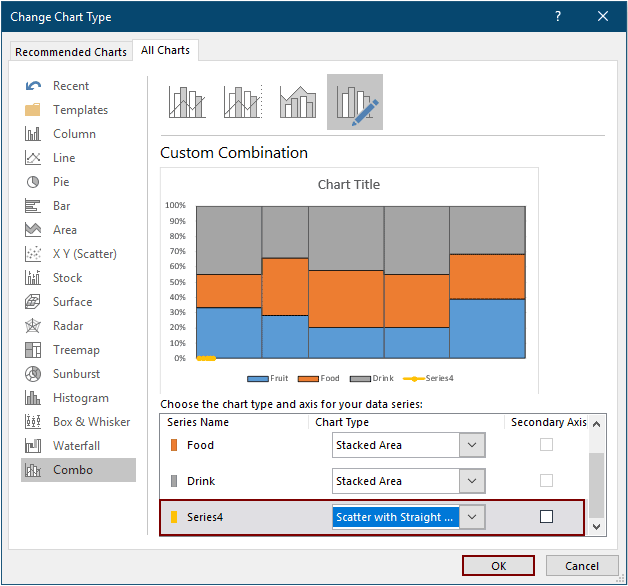17. Right click the chart and choose Select Data.

18. In the Select Data Source dialog box, select the Series4 (the series name you created in step 14) and click the Edit button in the Legend Entries (Series) box.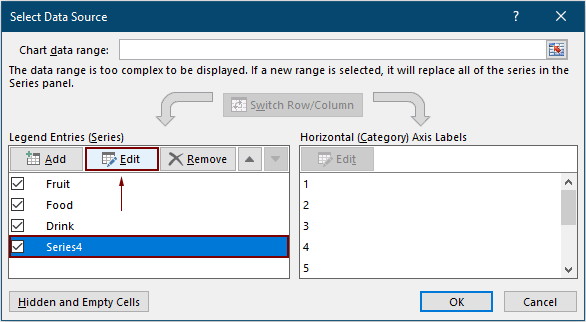19. In the Edit Series dialog box, select the first row cells in the Series X values box, and then click OK.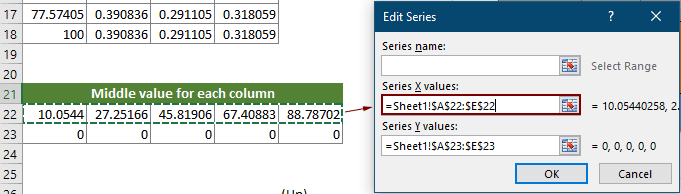20. Click OK to save the changes when it returns to the Select Data Source dialog box.

Now a new series is added at the bottom of the plot area as the below screenshot shown.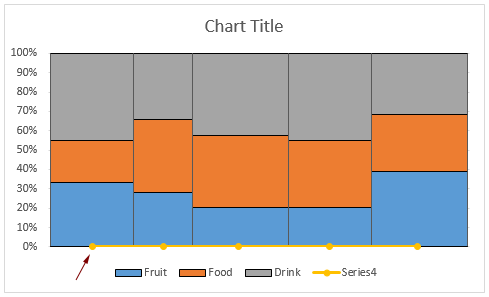21. Now you need to hide the line and markers. Please select this series, go to the Format Data Series pane and then do as follows.

21.1) Click the Fill & Line icon;
21.2) Select No line under the Line tab;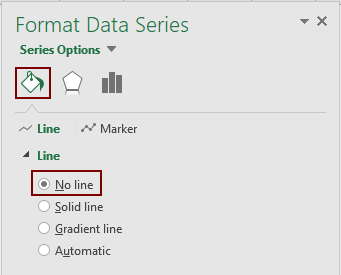21.3) Click the Marker tab, expand the Marker Options, and then select None.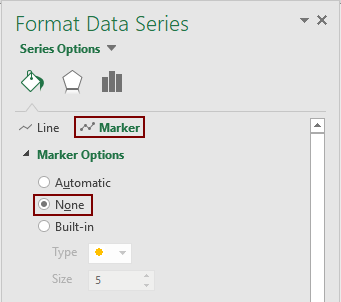22. Keep the series selected, click the Chart Elements button, and then check the Data Labels box.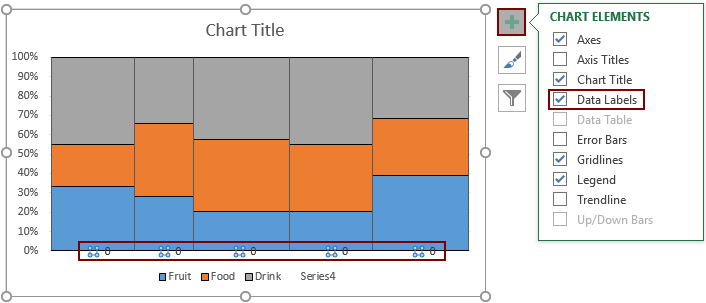23. Select the added labels, go to the Format Data Labels pane and configure as follows.

23.1) Check the Value From Cells box;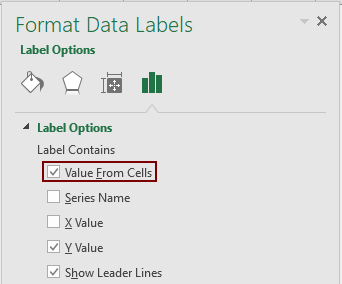23.2) In the Data Label Range dialog box, select the cells you want to display as the X-axis value and then click OK. Here I select the first column cells of the original data range.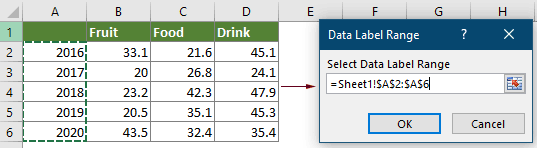23.3) Go back to the Format Data Labels pane, uncheck the Y Value box in the Label Contains section, and in the Label Position section, select the Below option.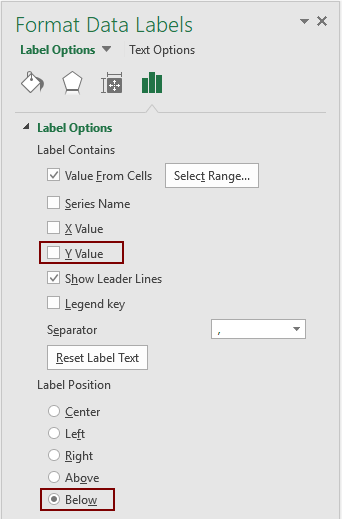Now the chart is displayed as follows.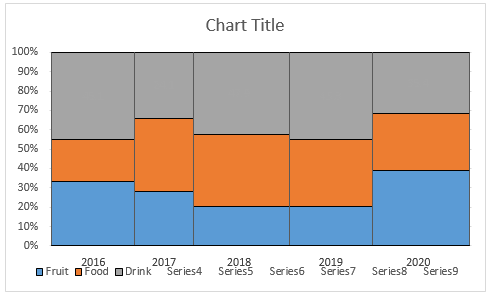##### Part4: Display one set of percentages above the column widths

Now we need to display one set of percentages above the column widths. Firstly, we need to calculate the percentages of each column.

24. As there are five columns in the chart, you need to calculate five percentages as follows.

24.1) Select a blank cell in a new row (here I select A27), enter the below formula into it and press the Enter key to get the percentage of the first column.
=\$A\$11/100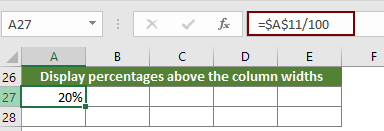24.2) For the second percentage, please apply the below formula.
=(A13-A11)/100
24.3) For the third percentage, apply the below formula.
=(A15-A13)/100
24.4) For the fourth percentage, apply the below formula.
=(A17-A15)/100
24.5) For the fifth percentage, apply the below formula.
=(A18-A17)/100

25. In the next row of the percentage, enter number 1 into each cell. Then you will get a new helper range as follows.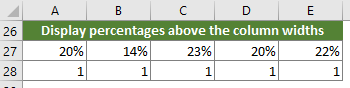26. Right click the chart and select Select Data from the right-clicking menu.

27. In the Select Data Source dialog box, click the Add button.

28. In the opening Edit Series dialog box, you need to do as follows.

28.1) In the Series X values box, select the cells containing the middle value for each column you have calculated in step 10.
Note: Here the middle values are used for displaying the percentages in the middle of the column widths.
28.2) In the Series Y values box, select the cells containing number 1.
Note: As the minimum and maximum of the Y-axis are 0 and 1, and we need to display the percentages above the plot area, here the Y value should be 1.
28.3) Click OK.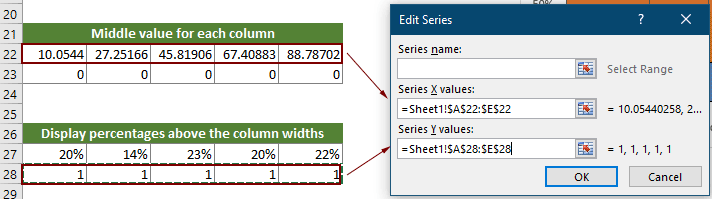29. When it returns to the Select Data Source dialog box, a new series (Series5) is created, click the OK button to save the changes.

30. Right click the chart and select Change Series Chart Type.

31. In the Change Chart Type dialog box, select the chart type “Scatter with Straight Lines and Markers” for the Series5 in the Choose the chart type and axis for your data series box. And then click OK.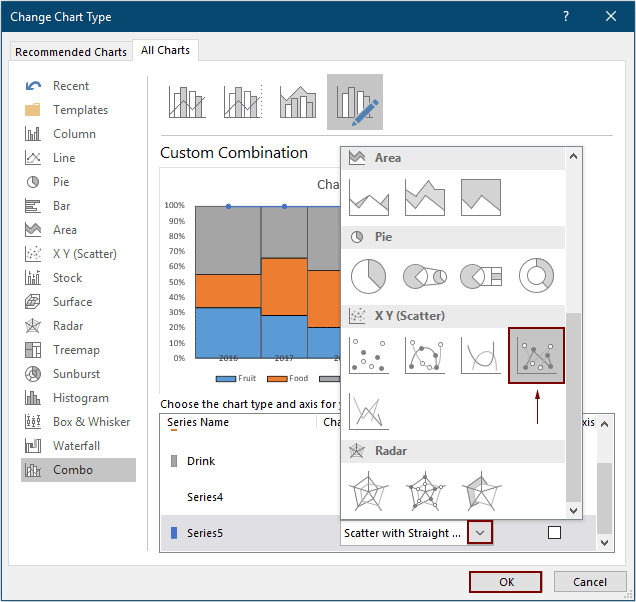Now the chart is displayed as follows.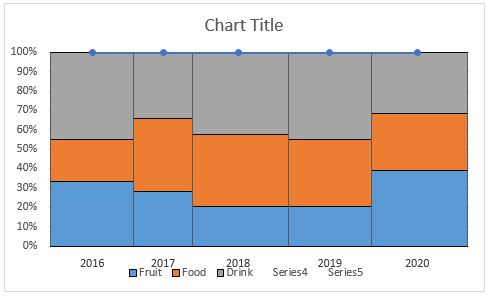32. You need to hide the line and markers of the series (Click to see how).

33. Add data labels to this series (Click to see how). Specify this label position to Above.

Note: In this section, after checking the Value From Cells box, you need to select the cells containing the calculated percentages in the Data Labels Range dialog box.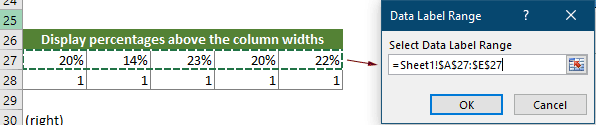Now the percentages are displayed above the column widths as the below screenshot shown.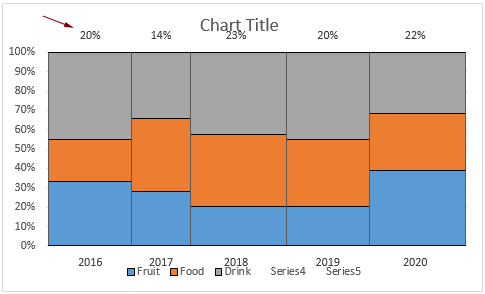##### Part5: Display the series names on the right of the plot area

As the below screenshot shown, for showing the series names on the right of the plot area in the chart, you need to calculate the middle values for each series of the last column firstly, add a new series based on this values and finally add the series names as the data labels of this new series.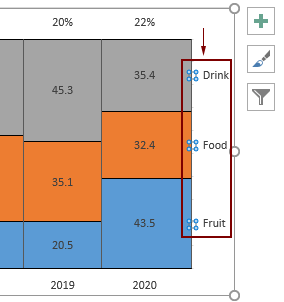34. To calculate the middle values for each series of the last column, please apply the below formulas.

34.1) Select a blank cell (here I select A31) in a new row, enter the below formula into it and press the Enter key.
=B6/SUM(B6:D6)/2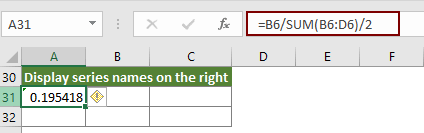34.2) Select the next cell (B31), enter the below formula into it and press the Enter key. Select this result cell and then drag its AutoFill Handle right to the next cell C31.
=SUM(\$B\$6:C6)/SUM(\$B\$6:\$D\$6)-C6/SUM(\$B\$6:\$D\$6)/2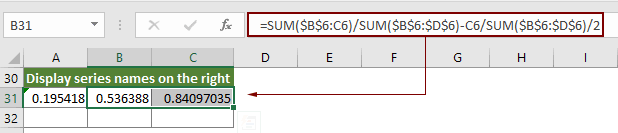35. In the next new row, enter number 100 into each cell. Finally another new helper range is created as the below screenshot shown.

Note: Here number 100 represents the maximum of the X-axis.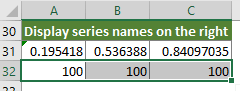36. Right click the chart and select Select Data from the context menu.

37. In the Select Data Source dialog box, click the Add button.

38. In the Edit Series dialog box, please select the corresponding range as follows.

38.1) In the Series X values box, select the cells containing number 100;
38.2) In the Series Y values box, select the cells containing the middle values for each series of the last column you have calculated in step 34.
38.3) Click the OK button.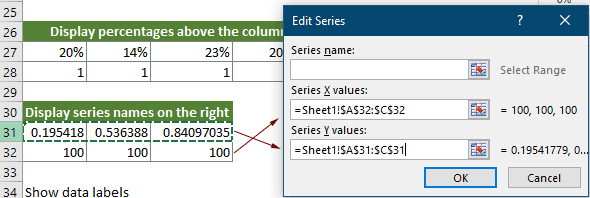39. When it returns to the Select Data Source dialog box, click OK to save the changes.

40. Right click the chart and select Change Series Chart Type from the context menu.

41. In the Change Chart Type dialog box, select the chart type “Scatter with Straight Lines and Markers” for the Series6 in the Choose the chart type and axis for your data series box, and then click OK.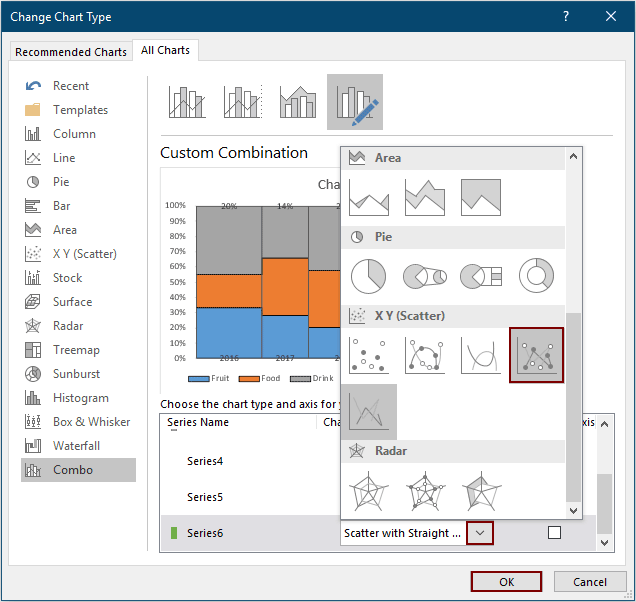Then a new series is added on the chart as the below screenshot shown.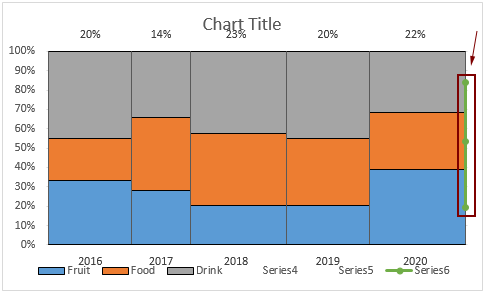42. You need to hide the line and markers of the series (Click to see how).

43. Add data labels to this series (Click to see how). Keep the Label Position as Right.

Note: In this section, after checking the Value From Cells box, you need to select the cells containing the series names in the original data range in the Data Labels Range dialog box.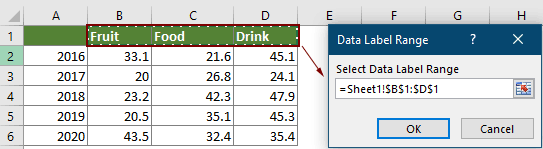Now the chart is displayed as follows.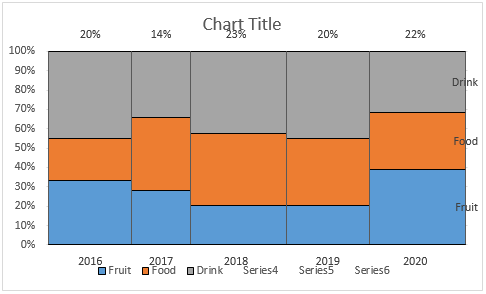##### Part6: Display the series values on each segment in columns

The last part here is going to show you how to display the series values(data labels) on each segment in columns as the below screenshot shown. Please do as follows.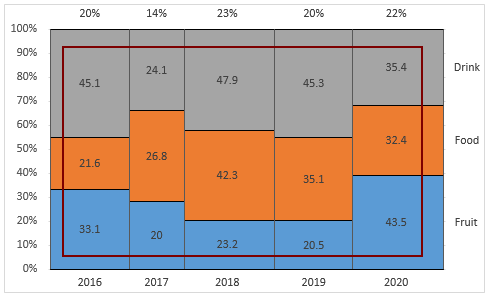44. Firstly, you need to calculate the middle value for each segment in columns, please apply the below formulas.

44.1) Starting with a new row, select the first blank cell (such as A35), enter the below formula into it and press the Enter key. Select this result cell and drag its AutoFill handle down to the below four cells.
Note: The result values in this column represent the middle values for the segments of Fruit series in each year.
=B2/SUM(B2:D2)/2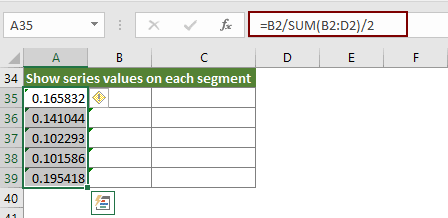44.2) Select the next cell B35, enter the below formula and press the Enter key. Then drag its AutoFill Handle down to the below four cells.
Note: The result values in this column represent the middle values for the segments of Food series in each year.
=C2/SUM(B2:D2)/2+Sheet1!B2/SUM(B2:D2)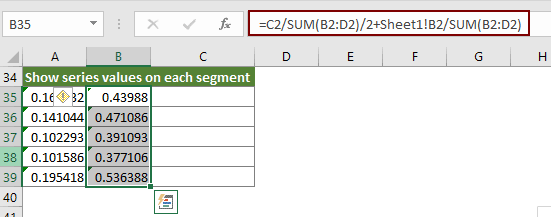44.3) Select C35, enter the below formula and press the Enter key. Then drag its AutoFill Handle down to the below four cells.
Note: The result values in this column represent the middle values for the segments of Drink series in each year.
=D2/SUM(B2:D2)/2+SUM(B2:C2)/SUM(B2:D2)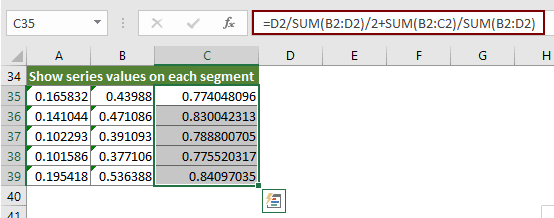45. Right click the chart and click Select Data in the context menu.

46. In the Select Data Source dialog box, click the Add button.

47. In the Edit Series dialog box, please select the corresponding ranges as follows.

47.1) In the Series X values box, select the cells containing the middle values for each column you have calculated in step 10.
Note: These values are used to determine the positions of the X-axis for the Fruit series.
47.2) In the Series Y values box, select the first column of the helper range you have created in this section.
Note: These values are used to determine the position of the Y-axis for the Fruit series.
47.3) Click the OK button.48. Repeat the step 46 and 47, using the remaining two column values to add two new series. See the below screenshots: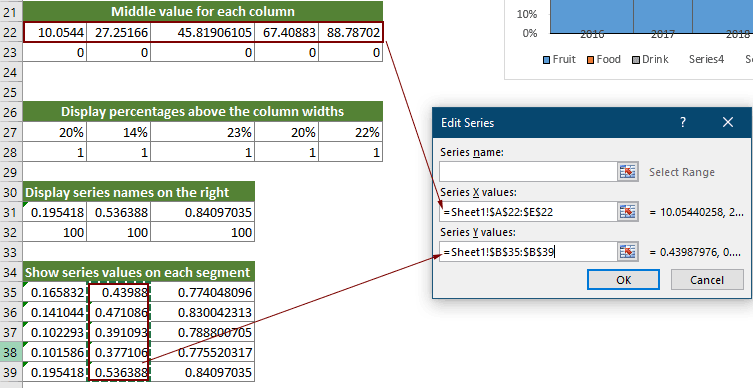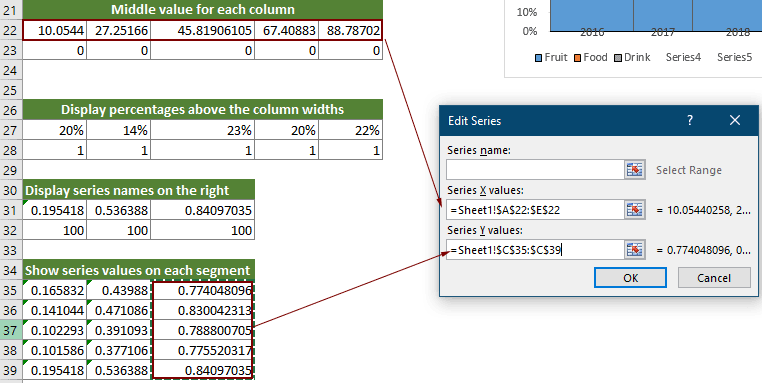49. When it returns to the Select Data Source dialog box, you can see three new series are added, click OK to save the changes.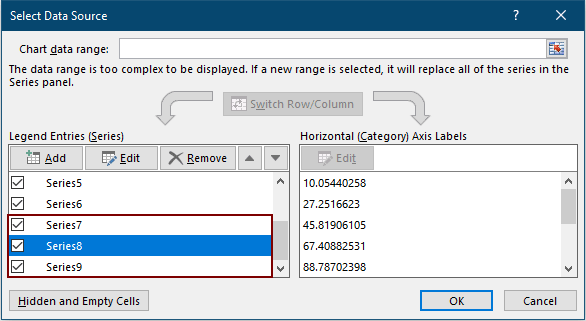50. Right click the chart and select Change Series Chart Type from the context menu.

51. In the Change Chart Type dialog box, separately select the chart type “Scatter with Straight Lines and Markers” for the these three new series in the Choose the chart type and axis for your data series box, and then click OK.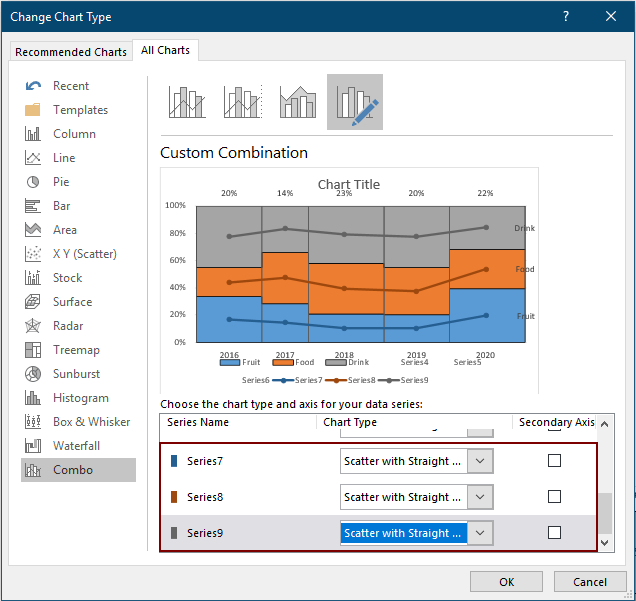The chart is displayed as follows.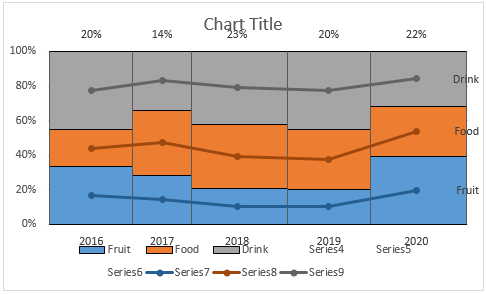52. You need to separately hide the lines and markers of the series (Click to see how).

53. Add data labels to the series (Click to see how). Specify the Label Position as Center.

Notes:

1) For the data labels of the Drink series, after checking the Value From Cells box, you need to select the actual values of the Drink series in the original data range.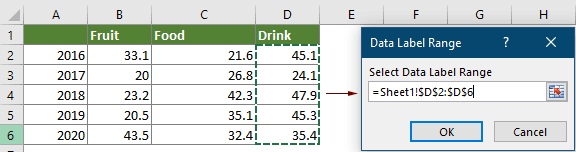2) For the data labels of the Food series, after checking the Value From Cells box, you need to select the actual values of the Food series in the original data range.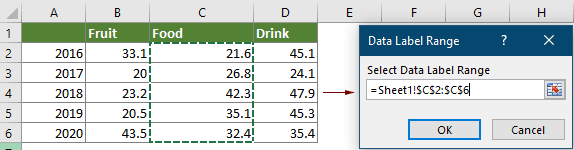3) For the data labels of the Fruit series, after checking the Value From Cells box, you need to select the actual values of the Fruit series in the original data range.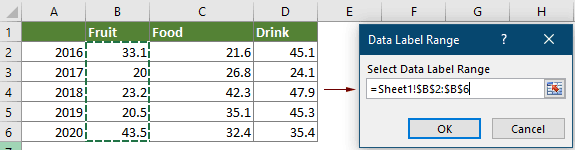Now the chart is displayed as the below screenshot shown.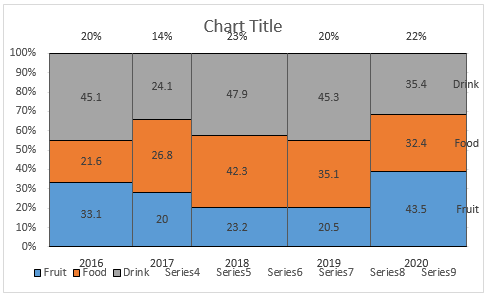54. Remove the chart title and the legend from the chart.

55. Keep the chart selected, go to the Format Data Series pane, and then select Plot Area in the Series Options drop-down list.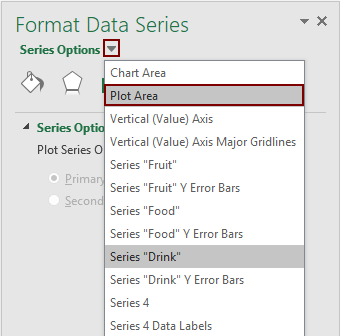56. The plot area of the chart is selected. Please narrow the plot area by dragging the borders until the above, bottom and the right values are fully displayed out of the plot area. See below demo.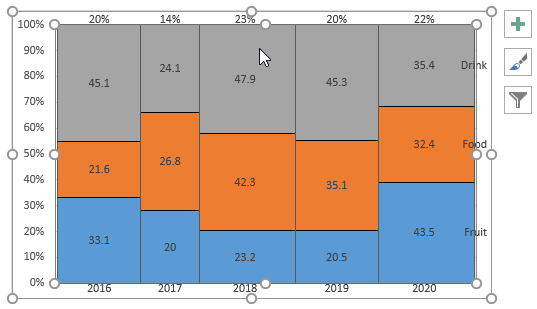Now a Marimekko Chart is complete.#### Easily create a marimekko chart in Excel

The Marimekko Chart utility of Kutools for Excel can help you quickly create a marimekko chart in Excel with several clicks only as the below demo shown.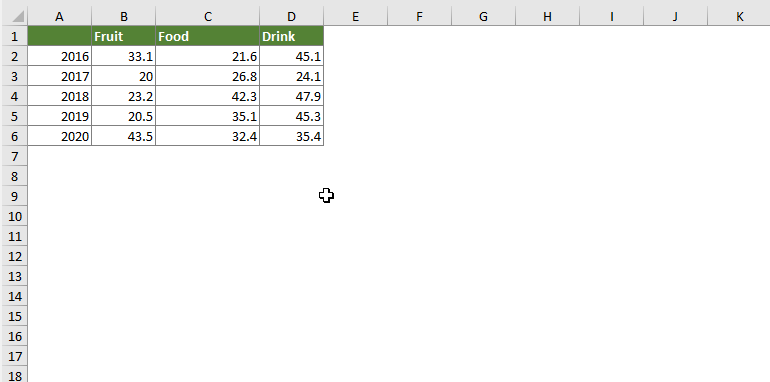### The Best Office Productivity Tools

#### Kutools for Excel - Helps You To Stand Out From Crowd

 Popular Features: Find, Highlight or Identify Duplicates  |  Delete Blank Rows  |  Combine Columns or Cells without Losing Data  |  Round without Formula ... Super VLookup: Multiple Criteria  |  Multiple Value  |  Across Multi-Sheets  |  Fuzzy Lookup... Adv. Drop-down List: Easy Drop Down List  |  Dependent Drop Down List  |  Multi-select Drop Down List... Column Manager: Add a Specific Number of Columns  |  Move Columns  |  Toggle Visibility Status of Hidden Columns  |  Compare Columns to Select Same & Different Cells ... Featured Features: Grid Focus  |  Design View  |  Big Formula Bar  |  Workbook & Sheet Manager | Resource Library (Auto Text)  |  Date Picker  |  Combine Worksheets  |  Encrypt/Decrypt Cells  |  Send Emails by List  |  Super Filter  |  Special Filter (filter bold/italic/strikethrough...) ... Top 15 Toolsets:  12 Text Tools (Add Text, Remove Characters ...)  |  50+ Chart Types (Gantt Chart ...)  |  40+ Practical Formulas (Calculate age based on birthday ...)  |  19 Insertion Tools (Insert QR Code, Insert Picture from Path ...)  |  12 Conversion Tools (Numbers to Words, Currency Conversion ...)  |  7 Merge & Split Tools (Advanced Combine Rows, Split Excel Cells ...)  |  ... and more

Kutools for Excel Boasts Over 300 Features, Ensuring That What You Need is Just A Click Away...#### Office Tab - Enable Tabbed Reading and Editing in Microsoft Office (include Excel)

• One second to switch between dozens of open documents!
• Reduce hundreds of mouse clicks for you every day, say goodbye to mouse hand.
• Increases your productivity by 50% when viewing and editing multiple documents.
• Brings Efficient Tabs to Office (include Excel), Just Like Chrome, Edge and Firefox.